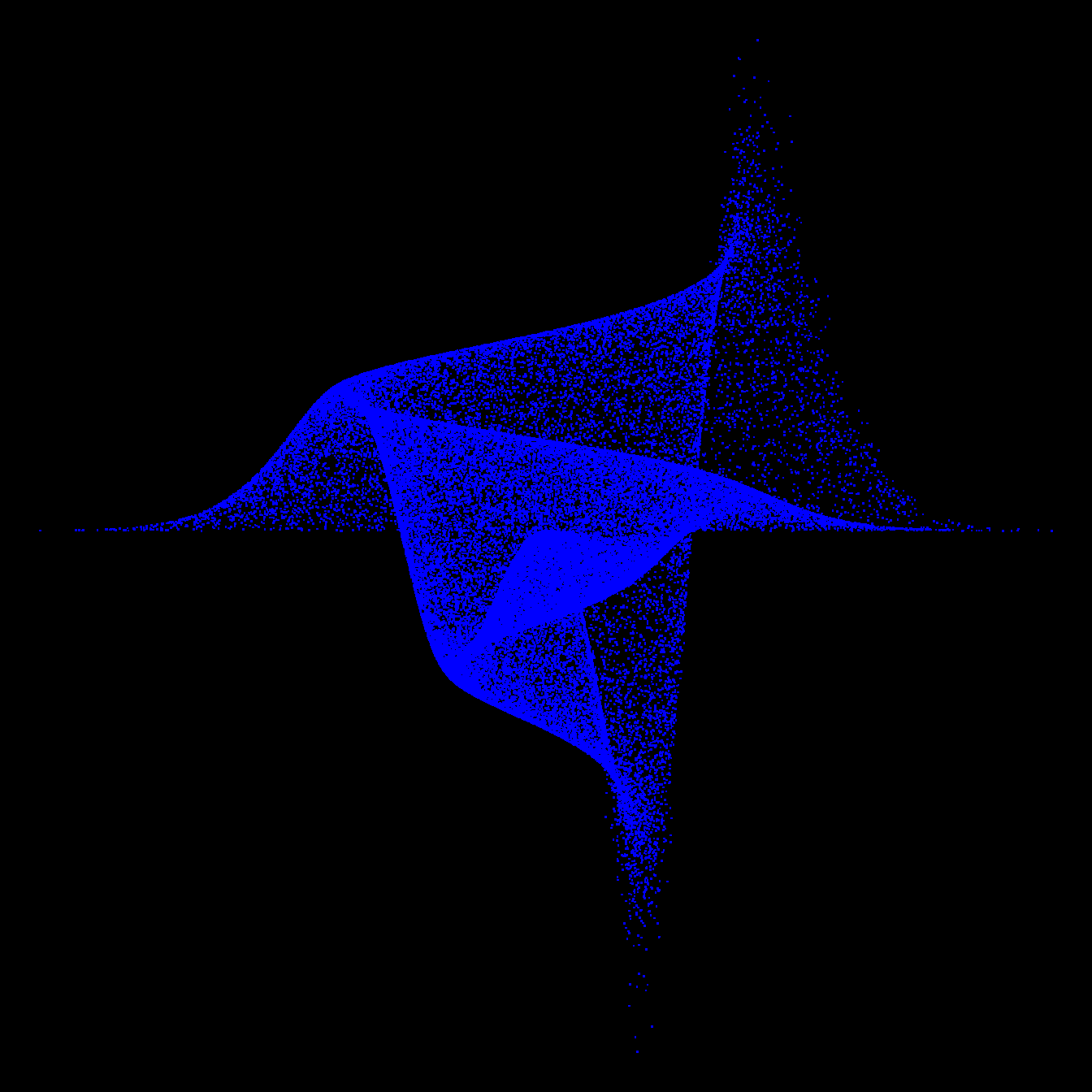# Nifty graph: a 3d imitation with R

A graph by Matt Asher showing probability function in a data art fashion.

This graph comes from the probability and statistics blog of Matt Asher Thanks for this contribution !``````moxbuller = function(n) {
u = runif(n)
v = runif(n)
x = cos(2*pi*u)*sqrt(-2*log(v))
y = sin(2*pi*v)*sqrt(-2*log(u))
r = list(x=x, y=y)
return(r)
}
r = moxbuller(50000)
par(bg="black")
par(mar=c(0,0,0,0))
plot(r\$x,r\$y, pch=".", col="blue", cex=1.2)``````

Related chart types

## Contact

This document is a work by Yan Holtz. Any feedback is highly encouraged. You can fill an issue on Github, drop me a message on Twitter, or send an email pasting yan.holtz.data with gmail.com.